## Questions

1. P varies as the square of R. R. varies as the square of T. When P = 18, R = 3 and T = 5. Express P in terms of T hence find P when T = 10.
2. Make r the subject of the formula.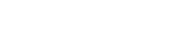3. X varies as the cube of Y and inversely as square root of Z, X = 6 when Y = 3 and Z= 25.
1. Find;
1. An expression connecting X,Y,Z
2. X when Y = 7 and Z = 9
3. Y when X = 8 and Z = 16
2. If Y is increased by 20% and Z is decreased by 36%, find the percentage increase in X
4. Make b the subject of the formula;
K = ab
b –a
5. A quantity Z varies directly as the square of x and inversely as the square root of y. If x increases by 20% and y decreases by 36%, find the percentage change in Z.
6. A quantity P varies directly as the square of Q and inversely as quantity R. If P = 2 when Q = 4 and R=6, find P when Q = 8 and R= 4.
7. B varies partly as the square of M and partly as the inverse of N. B,M and N are such that when M=2, N= ½ , B=96 while when M= 3 , N=2, B = 46. Write an expression for B in terms of M and N.
8. Solve for x and y.
3x    = 1
y - 1
(2x + 2) : (y – 5) = 1 : 2
9. Make x the subject of the formula.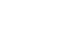10. Make d the subject of the formula given that:-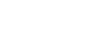11. Z varies jointly as the square of x and inversely as the square of y. When x = 10 and
y = 4 then z = 15.
1. Find z in terms of x and y
2. Find the value of x when z = 8 and y = 12
12. A quantity R partly varies as n and partly as the square root of n. When n = 9 R = 42 and when n = 25 R = 100. Find R when n = 16.
13. Make b the subject of the formula.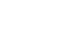14. P varies party as Q and partly as the square root of Q. When Q = 4, P = 22 and when
Q = 9, P = 42. Find the value of P when Q = 25.
15. Make C the subject of the formula
b = √(k-aC)
hence find the value of C when K= 1, a = 4 and b = 2
16. The velocity of water flowing through a pipe is inversely proportional to the square of the
radius of the pipe. If the velocity of the water is 30cm/s when the radius of the pipe is 2cm. Find the velocity of water when the radius of the pipe is 4cm.
17. Make x the subject of the formula.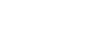18. Three quantities x, y and z are such that x varies partly as y and partly as the inverse
of the square of Z . When x = 6, y = 3 and z= 2. When x = 8, y = 5 and z= 1. Find the
value of x when y = 10 and z= 8.
19. The resistance of an electrical conductor is partly constant and partly varies as the temperature. When the temperature is 20oC, the resistance is 55 ohms. When the temperature is 28oC, the resistance is 58 ohms. Find the resistance when the temperature is 60oC

1.2.3.4. K ( b-a) = ab
Kb – ka = ab
Kb – ab = ka
B(k-a) = ka
B =    ka
K –a

5.6.7.8.9.10.11.12.13.14. P = KQ + m√Q
22 = K (4) + m(2)……………(1)
42 = K(g) + n(3)……………(2)
22= 4K + 2m
42 = 9K + 3m
3(22) = 3(4K) + 3(2m)
2(42) = 2(9K) + 2(3)
66 = 12k + 6m
84 = 18K + 6m
18= 6k = k=3
22 = 4(3) + 2m
22-12 = 2m
20 = 2m
M = 10
= 3(25) + 10(5)
= 75 + 50
= 125

15.16. V = 30, r = 2
K = Ur2
= 30 x 22 = 120
When r = 4
V = 120/42 = 7.5m/s

17.18.19. R = m + nI
55 = M + 20n……(i)
58 = m + 28n…….(ii)
-3 = -8n
n = 3/8 = 0.375
55 = m + 60/8
m =55 -7.5 => m = 47.5
R = 47. 5 + 60 X 3/8
R = 70 ohms

#### Download Formulae and variation Questions and Answers - Form 3 Topical Mathematics.

• ✔ To read offline at any time.
• ✔ To Print at your convenience
• ✔ Share Easily with Friends / Students

Read 9454 times Last modified on Wednesday, 10 February 2021 07:00
.
Subscribe now

access all the content at an affordable rate
or
Buy any individual paper or notes as a pdf via MPESA
and get it sent to you via WhatsApp